# cghfreqplot

Display frequency of DNA copy number alterations across multiple samples

## Syntax

```FreqStruct = cghfreqplot(CGHData) FreqStruct = cghfreqplot(CGHData, ...'Threshold', ThresholdValue, ...) FreqStruct = cghfreqplot(CGHData, ...'Group', GroupValue, ...) FreqStruct = cghfreqplot(CGHData, ...'Subgrp', SubgrpValue, ...) FreqStruct = cghfreqplot(CGHData, ...'Subplot', SubplotValue, ...) FreqStruct = cghfreqplot(CGHData, ...'Cutoff', CutoffValue, ...) FreqStruct = cghfreqplot(CGHData, ...'Chromosome', ChromosomeValue, ...) FreqStruct = cghfreqplot(CGHData, ...'IncludeX', IncludeXValue, ...) FreqStruct = cghfreqplot(CGHData, ...'IncludeY', IncludeYValue, ...) FreqStruct = cghfreqplot(CGHData, ...'Chrominfo', ChrominfoValue, ...) FreqStruct = cghfreqplot(CGHData, ...'ShowCentr', ShowCentrValue, ...) FreqStruct = cghfreqplot(CGHData, ...'Color', ColorValue, ...) FreqStruct = cghfreqplot(CGHData, ...'YLim', YLimValue, ...) FreqStruct = cghfreqplot(CGHData, ...'Titles', TitlesValue, ...) ```

## Input Arguments

 `CGHData` Array-based comparative genomic hybridization (aCGH) data in either of the following forms:Structure with the following fields:`Sample` — Cell array of character vectors or string vector containing the sample names (optional).`Chromosome` — Vector containing the chromosome numbers on which the clones are located.`GenomicPosition` — Vector containing the genomic positions (in bp, kb, or mb units) to which the clones are mapped.`Log2Ratio` — Matrix containing log2 ratio of test to reference signal intensity for each clone. Each row corresponds to a clone, and each column corresponds to a sample.Matrix in which each row corresponds to a clone. The first column contains the chromosome number, the second column contains the genomic position, and the remaining columns each contain the log2 ratio of test to reference signal intensity for a sample. `ThresholdValue` Positive scalar or vector that specifies the gain/loss threshold. A clone is considered to be a gain if its log2 ratio is above `ThresholdValue`, and a loss if its log2 ratio is below negative `ThresholdValue`. The `ThresholdValue` is applied as follows:If a positive scalar, it is the gain and loss threshold for all the samples. If a two-element vector, the first element is the gain threshold for all samples, and the second element is the loss threshold for all samples.If a vector of the same length as the number of samples, each element in the vector is considered as a unique gain and loss threshold for each sample. Default is `0.25`. `GroupValue` Specifies the sample groups to calculate the frequency from. Choices are:A vector of sample column indices (for data with only one group). The samples specified in the vector are considered a group.A cell array of vectors of sample column indices (for data divided into multiple groups). Each element in the cell array is considered a group.Default is a single group of all the samples in `CGHData`. `SubgrpValue` Controls the analysis of samples by subgroups. Choices are `true` (default) or `false`. `SubplotValue` Controls the display of all plots in one Figure window when more than one subgroup is analyzed. Choices are `true` (default) or `false` (displays plots in separate windows). `CutoffValue` Scalar or two-element numeric vector that specifies a cutoff, which controls the plotting of only the clones with frequency gains or losses greater than or equal to `CutoffValue`. If a two-element vector, the first element is the cutoff for gains, and the second element is for losses. Default is `0`. `ChromosomeValue` Single chromosome number or a vector of chromosome numbers that specify the chromosomes for which to display frequency plots. Default is all chromosomes in `CGHData`. `IncludeXValue` Controls the inclusion of the X chromosome in the analysis. Choices are `true` (default) or `false`. `IncludeYValue` Controls the inclusion of the Y chromosome in the analysis. Choices are `true` or `false` (default) . `ChrominfoValue` Cytogenetic banding information specified by either of the following:Structure returned by the `cytobandread` functionCharacter vector or string specifying the file name of an NCBI ideogram text file or a UCSC Genome Browser cytoband text fileDefault is Homo sapiens cytogenetic banding information from the UCSC Genome Browser, NCBI Build 36.1 (`https://genome.UCSC.edu`). `ShowCentrValue` Controls the display of the centromere positions as vertical dashed lines in the frequency plot. Choices are `true` (default) or `false`.TipThe centromere positions are obtained from `ChrominfoValue`. `ColorValue` Color scheme for the vertical lines in the plot, indicating the frequency of the gains and losses, specified by either of the following:Name of or handle to a function that returns a colormapM-by-3 matrix containing RGB values. If M equals 1, then that single color is used for all gains and losses. If M equals 2 or more, then the first row is used for gains, the second row is used for losses, and remaining rows are ignored. For example, ```[0 1 0;1 0 0]``` specifies green for gain and red for loss. The default color scheme is a range of colors from pure green (gain = 1) through yellow (0) to pure red (loss = –1). `YLimValue` Two-element vector specifying the minimum and maximum values on the vertical axis. Default is `[1, -1]`. `TitlesValue` Character vector, string, string vector, or a cell array of character vectors that specifies titles for the group(s), which are added to the tops of the plot(s).

## Output Arguments

 `FreqStruct` Structure containing frequency data in the following fields:`Group` — Structure array, with each structure representing a group of samples. Each structure contains the following fields:`Sample` — Cell array containing names of samples within the group.`GainFrequency` — Column vector containing the average gain for each clone for a group of samples.`LossFrequency` — Column vector containing the average loss for each clone for a group of samples.`Chromosome` — Column vector containing the chromosome numbers on which the clones are located.`GenomicPosition` — Column vector containing the genomic positions of the clones.TipYou can use this output structure as input to the `cghfreqplot` function.

## Description

`FreqStruct = cghfreqplot(CGHData)` displays the frequency of copy number gain or loss across multiple samples for each clone on an array against their genomic position along the chromosomes.

```FreqStruct = cghfreqplot(CGHData, ...'PropertyName', PropertyValue, ...)``` calls `cghfreqplot` with optional properties that use property name/property value pairs. You can specify one or more properties in any order. Each `PropertyName` must be enclosed in single quotation marks and is case insensitive. These property name/property value pairs are as follows:

``` FreqStruct = cghfreqplot(CGHData, ...'Threshold', ThresholdValue, ...)``` specifies the gain/loss threshold. A clone is considered to be a gain if its log2 ratio is above `ThresholdValue`, and a loss if its log2 ratio is below negative `ThresholdValue`.

The `ThresholdValue` is applied as follows:

• If a positive scalar, it is the gain and loss threshold for all the samples.

• If a two-element vector, the first element is the gain threshold for all samples, and the second element is the loss threshold for all samples.

• If a vector of the same length as the number of samples, each element in the vector is considered as a unique gain and loss threshold for each sample.

Default is `0.25`.

```FreqStruct = cghfreqplot(CGHData, ...'Group', GroupValue, ...)``` specifies the sample groups to calculate the frequency from. Choices are:

• A vector of sample column indices (for data with only one group). The samples specified in the vector are considered a group.

• A cell array of vectors of sample column indices (for data divided into multiple groups). Each element in the cell array is considered a group.

Default is a single group of all the samples in `CGHData`.

```FreqStruct = cghfreqplot(CGHData, ...'Subgrp', SubgrpValue, ...)``` controls the analysis of samples by subgroups. Choices are `true` (default) or `false`.

```FreqStruct = cghfreqplot(CGHData, ...'Subplot', SubplotValue, ...)``` controls the display of all plots in one Figure window when more than one subgroup is analyzed. Choices are `true` (default) or `false` (displays plots in separate windows).

```FreqStruct = cghfreqplot(CGHData, ...'Cutoff', CutoffValue, ...)``` specifies a cutoff value, which controls the plotting of only the clones with frequency gains or losses greater than or equal to `CutoffValue`. `CutoffValue` is a scalar or two-element numeric vector. If a two-element numeric vector, the first element is the cutoff for gains, and the second element is for losses. Default is `0`.

```FreqStruct = cghfreqplot(CGHData, ...'Chromosome', ChromosomeValue, ...)``` displays the frequency plots only of chromosome(s) specified by `ChromosomeValue`, which can be a single chromosome number or a vector of chromosome numbers. Default is all chromosomes in `CGHData`.

```FreqStruct = cghfreqplot(CGHData, ...'IncludeX', IncludeXValue, ...)``` controls the inclusion of the X chromosome in the analysis. Choices are `true` (default) or `false`.

```FreqStruct = cghfreqplot(CGHData, ...'IncludeY', IncludeYValue, ...)``` controls the inclusion of the Y chromosome in the analysis. Choices are `true` or `false` (default).

```FreqStruct = cghfreqplot(CGHData, ...'Chrominfo', ChrominfoValue, ...)``` specifies the cytogenetic banding information for the chromosomes. `ChrominfoValue` can be either of the following

• Structure returned by the `cytobandread` function

• Character vector or string specifying the file name of an NCBI ideogram text file or a UCSC Genome Browser cytoband text file

Default is Homo sapiens cytogenetic banding information from the UCSC Genome Browser, NCBI Build 36.1 (`https://genome.UCSC.edu`).

Tip

You can download files containing cytogenetic G-banding data from the NCBI or UCSC Genome Browser web site. For example, you can download the cytogenetic banding data (`cytoBandIdeo.txt.gz`) for Homo sapiens from:

`https://hgdownload.cse.ucsc.edu/goldenPath/hg19/database/`

```FreqStruct = cghfreqplot(CGHData, ...'ShowCentr', ShowCentrValue, ...)``` controls the display of the centromere positions as vertical dashed lines in the frequency plot. Choices are `true` (default) or `false`.

Tip

The centromere positions are obtained from `ChrominfoValue`.

```FreqStruct = cghfreqplot(CGHData, ...'Color', ColorValue, ...)``` specifies a color scheme for the vertical lines in the plot, indicating the frequency of the gains and losses. Choices are:

• Name of or handle to a function that returns a colormap.

• M-by-3 matrix containing RGB values. If M equals 1, then that single color is used for all gains and losses. If M equals 2 or more, then the first row is used for gains, the second row is used for losses, and remaining rows are ignored. For example, ```[0 1 0;1 0 0]``` specifies green for gain and red for loss.

The default color scheme is a range of colors from pure green (gain = 1) through yellow (0) to pure red (loss = –1).

```FreqStruct = cghfreqplot(CGHData, ...'YLim', YLimValue, ...)``` specifies the y vertical limits for the frequency plot. `YLimValue` is a two-element vector specifying the minimum and maximum values on the vertical axis. Default is `[1, -1]`.

```FreqStruct = cghfreqplot(CGHData, ...'Titles', TitlesValue, ...)``` specifies titles for the group(s), which are added to the tops of the plot(s). `TitlesValue` can be a character vector, string, string vector, or a cell array of character vectors.

## Examples

collapse all

Plot data from the Coriell cell line study

Load the array-based CGH (aCGH) data from the Coriell cell line study (Snijders, A. et al., 2001).

`load coriell_baccgh`

Display a frequency plot of the copy number alterations across all samples.

`Struct = cghfreqplot(coriell_data);`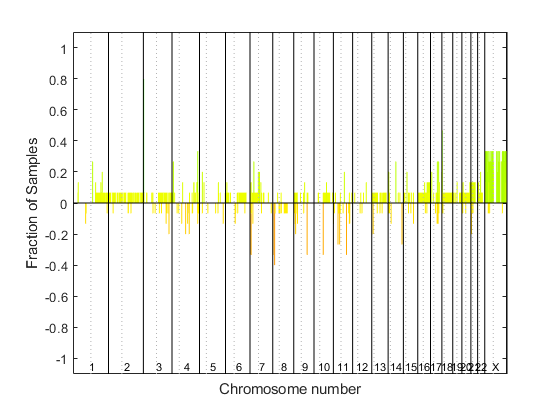View data tips for the data, chromosomes, and centromeres. First click the Data Cursor button on the toolbar, then click the black chromosome boundary line, or a dotted centromere line in the plot. To delete this data tip, right-click it, then select Delete Current Datatip.

Display a color bar indicating the degree of gain or loss by clicking the Insert Colorbar button on the toolbar.

Plot data from a pancreatic cancer study

Load the aCGH data from a pancreatic cancer study (Aguirre, A. et al., 2004).

`load pancrea_oligocgh`

Display a frequency plot of the copy number alterations across all samples using a green and red color scheme.

`cghfreqplot(pancrea_data, 'Color', [0 1 0; 1 0 0])`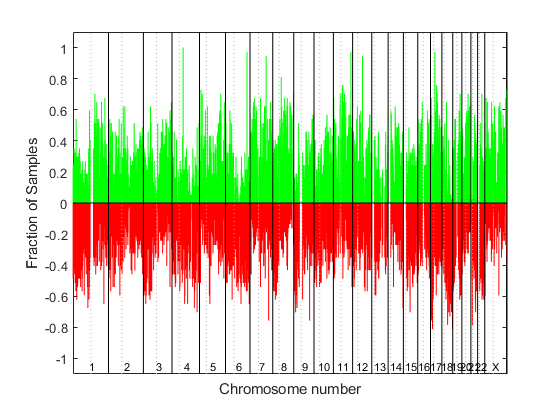Plotting groups of aCGH Data

Define two groups of data.

```grp1 = strncmp('PA.C', pancrea_data.Sample,4); grp1_ind = find(grp1); grp2 = strncmp('PA.T', pancrea_data.Sample,4); grp2_ind = find(grp2);```

Display a frequency plot of the copy number alterations across all samples in the two groups and limit the plotting to only the clones with frequency gains or losses greater than or equal to 0.25.

```SP = cghfreqplot(pancrea_data, 'Group', {grp1_ind, grp2_ind},... 'Title', {'CL', 'PT'}, 'Cutoff', 0.25);```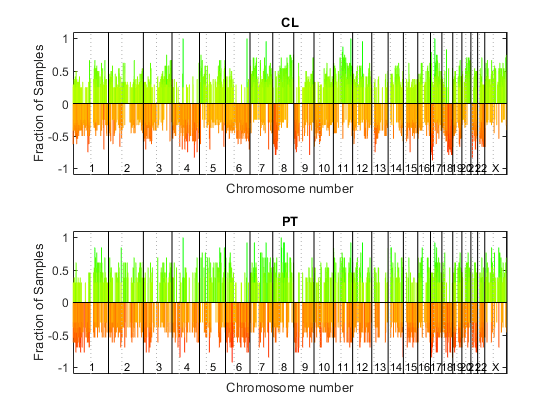Display a frequency plot of the copy number alterations across all samples in the first group and limit the plot to chromosome 4 only.

```SP = cghfreqplot(pancrea_data, 'Group', grp1_ind, ... 'Title', 'CL Group on Chr 4', 'Chromosome', 4);```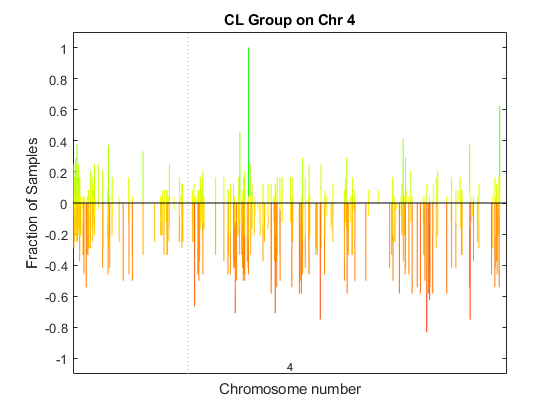Use the `chromosomeplot` function with the `'addtoplot'` option to add the ideogram of chromosome 4 for Homo sapiens to this frequency plot. Because the plot of the frequency data from the pancreatic cancer study is in kb units, use the `'Unit'` option to convert the ideogram data to kb units.

```fh = gcf; currentAxes = fh.CurrentAxes; chromosomeplot('hs_cytoBand.txt', 4, 'addtoplot', currentAxes, 'Unit', 2);```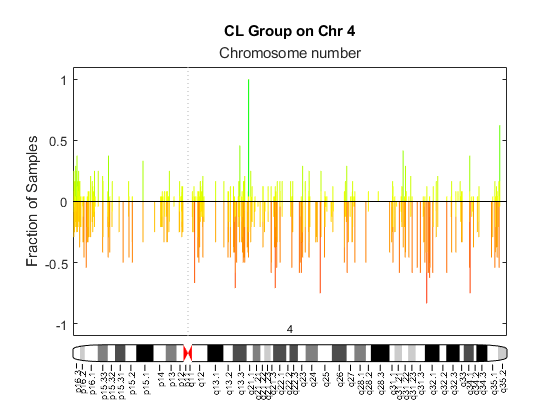## References

 Snijders, A.M., Nowak, N., Segraves, R., Blackwood, S., Brown, N., Conroy, J., Hamilton, G., Hindle, A.K., Huey, B., Kimura, K., Law, S., Myambo, K., Palmer, J., Ylstra, B., Yue, J.P., Gray, J.W., Jain, A.N., Pinkel, D., and Albertson, D.G. (2001). Assembly of microarrays for genome-wide measurement of DNA copy number. Nature Genetics 29, 263–264.

 Aguirre, A.J., Brennan, C., Bailey, G., Sinha, R., Feng, B., Leo, C., Zhang, Y., Zhang, J., Gans, J.D., Bardeesy, N., Cauwels, C., Cordon-Cardo, C., Redston, M.S., DePinho, R.A., and Chin, L. (2004). High-resolution characterization of the pancreatic adenocarcinoma genome. PNAS 101, 24, 9067–9072.

## Version History

Introduced in R2008a